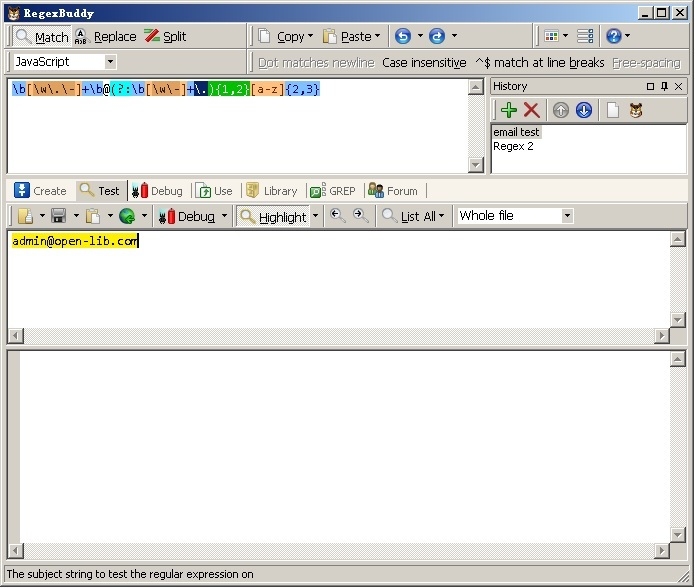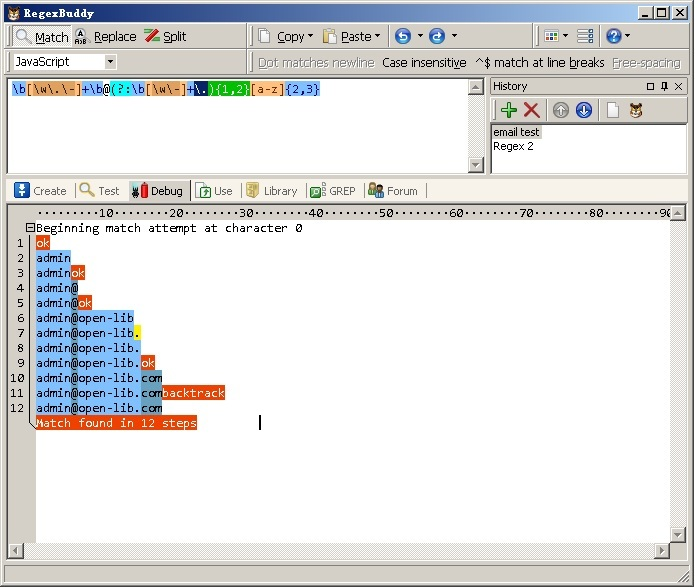# 正则表达式的优化---用RegexBuddy进行效率分析

^\w+([\.-]\w+)*@\w+([\.-]\w+)*\.\w+([-\.]\w+)*$ 该表达式有几个不足之处： 1. 头尾不应该用^$
2. 无须分组，这里用了三个组，若其用于网络爬虫将严重拖慢速度、吞噬内存
3. 没有域名合法性检查
4. @符号前名称判断太罗嗦
5. @符号后二处\w+([.-]\w+)*，明显太累赘

\b[\w\.\-]+\b@(?:\b[\w\-]+\.){1,2}[a-zA-Z]{2,3}\b[\w\.\-]+\b@\b[\w\-]+\b(?:\.[A-Za-z]{2,3}){1,2}\b